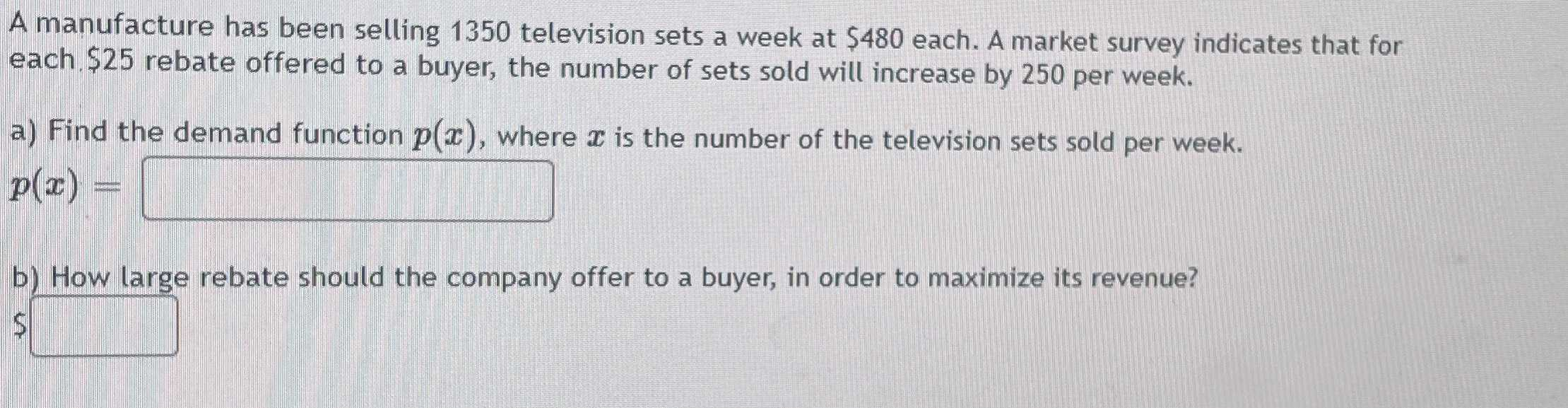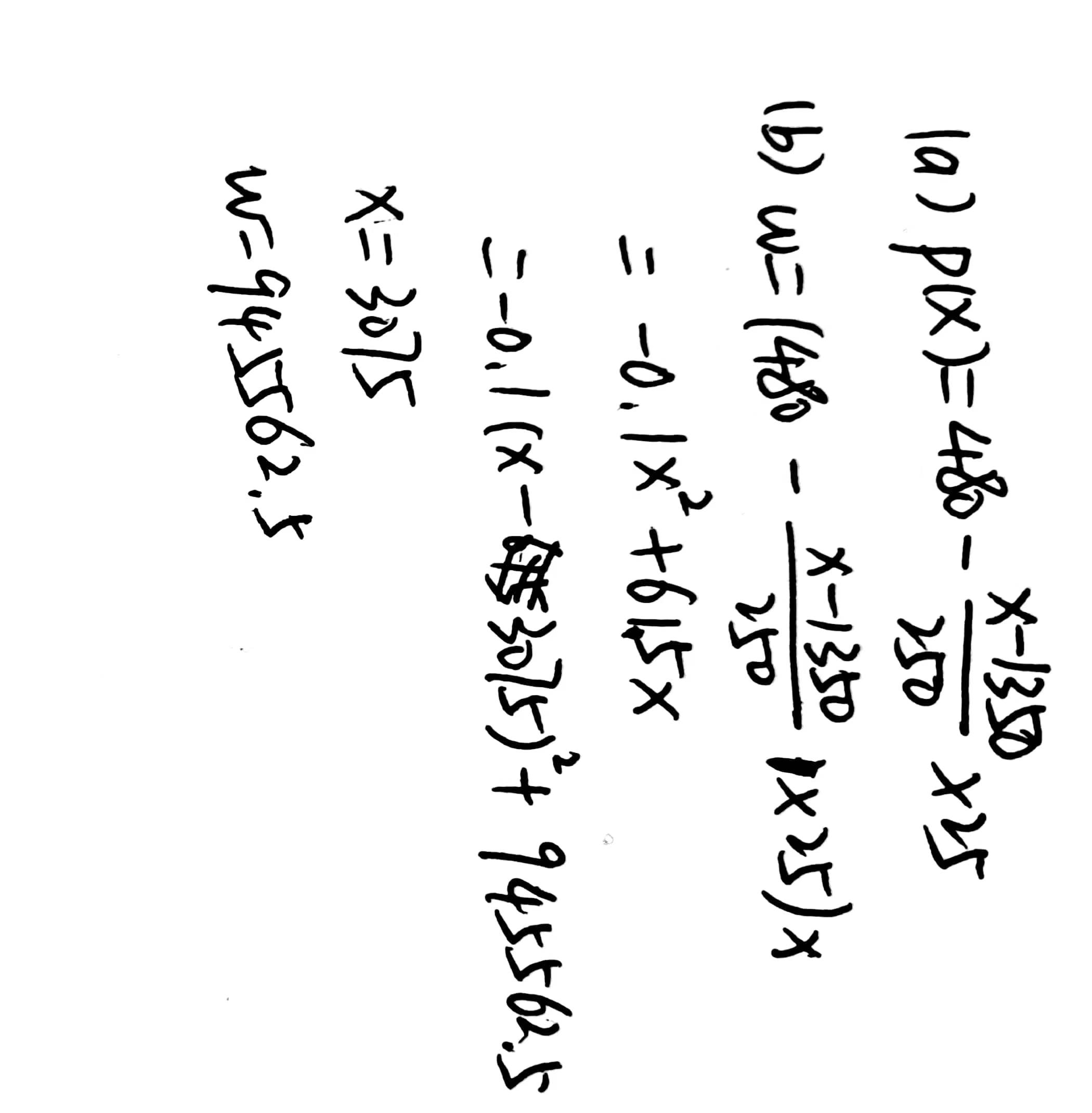### Still have math questions?

Algebra
QuestionA manufacture has been selling $$1350$$ television sets a week at $$\ 480$$ each. A market survey indicates that for each. $$\ 25$$ rebate offered to a buyer, the number of sets sold will increase by $$250$$ per week.

a) Find the demand function $$p ( x )$$ , where $$x$$ is the number of the television sets sold per week.

$$p ( x ) =$$

b) How large rebate should the company offer to a buyer, in order to maximize its revenue?

$$\$$